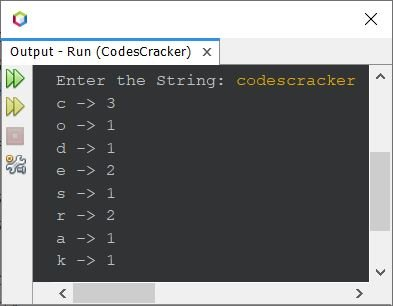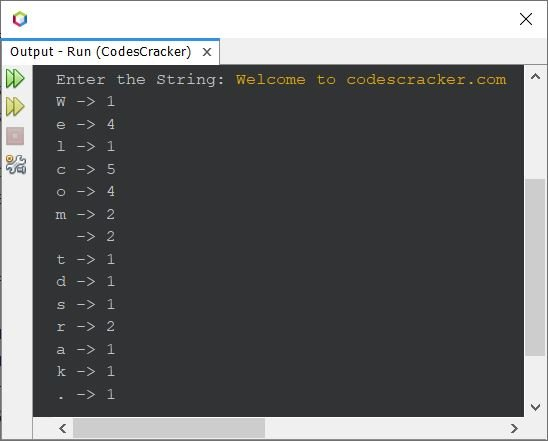# Java Program to Count the Occurrence of Each Character in String

This article is created to cover a program in Java, to count the occurrence of each character in a string entered by user at run-time of the program.

The question is, write a Java program to count and print the occurrence of each characters available in a string. The string must be received by user at run-time. The program given below is its answer:

```import java.util.Scanner;

public class CodesCracker
{
public static void main(String[] args)
{
int len, i, k, count, prevLen, arri=0, arrLen=0;
String str;
char ch;
Scanner s = new Scanner(System.in);

System.out.print("Enter the String: ");
str = s.nextLine();

len = str.length();
char[][] arr = new char[len];

for(i=0; i<len; i++)
{
ch = str.charAt(i);
count = 0;
for(k=0; k<arrLen; k++)
{
if(ch==arr[k])
{
prevLen = (arr[k]);
prevLen = prevLen+1;
arr[k] = (char)prevLen;
count++;
break;
}
}
if(count==0)
{
arr[arri] = ch;
arr[arri] = '1';
arrLen++;
arri++;
}
}
for(i=0; i<arrLen; i++)
System.out.println(arr[i]+ " -> " +arr[i]);
}
}```

Here is its sample run with user input codescracker as string to count occurrence of each character available in this given string:Here is another sample run with user input, Welcome to codescracker.com:In above sample output, in between the character m and t, the occurrence 2 refers to white space.

In above program, I've created a two dimensional array. That is, array inside array. The inner array is of size 2. Where at the first index, I've placed the character, and at second, I've placed its occurrence. And the whole array of character and its occurrence, is wrapped in an outer array of size equals to the total number of unique characters, available in given string.

Note - Since the array arr is of char data type, therefore before initializing the value of prevLen (an int type variable), we need to typecast the variable into char. So I've done using (char)prevLen.

The dry run of above program with user input codescracker goes like:

• Initial values, arri=0, arrLen=0, str="codescracker" (entered by user)
• Using the statement
`len = str.length();`
the length of entered string, that will be 12, will get initialized to len variable
• Now a character array named arr is declared, whose maximum size is 12. That is, the array arr can contain maximum 12 arrays of 2-elements each. It occupies its maximum size, only if all characters entered by user are unique. This array is created to store character and its occurrence.
• As soon as the occurrence increases, of any character, the size will get decreases. Because, we do not need to store that duplicate character, rather we need to increment the previous occurrence value by 1 more, of that character.
• Now the execution of for loop begins
• Initially i=0, and since the condition i<len or 0<12 evaluates to be True. therefore program flow goes inside the loop
• Using the statement
`ch = str.charAt(i);`
the character at index number i in str, will get initialized to ch
• Therefore str.charAt(0) or 'c' will get initialized to ch. And then using the next statement, 0 will get initialized to count variable. This variable is used to check whether the current character is available in the character array arr or not
• Now the execution of inner for loop begins
• Initially k=0 and the condition k<arrLen or 0<0 evaluates to be False. Therefore program flow does not goes inside this loop
• After this loop, the condition of if will gets evaluated. That is, the condition count==0 or 0==0 evaluates to be True. Therefore program flow goes inside the if's block and evaluates all the four statements available inside it
• So, ch or 'c' will get initialized to arr[arri] or arr. Similarly, arr='1', arrLen=1, arri=1 (by incrementing its value)
• Now the value of i will get incremented. That is i=1 and the condition i<len (condition of outer for loop), or 1<12 evaluates to be True again
• Therefore, program flow again goes inside the loop
• The next character, second character 'o' will get initialized to ch. And count=0 again
• The execution of inner loop begins, again
• That is, k=0 and k<arrLen or 0<1 evaluates to be True, therefore program flow goes inside this loop and the condition ch==arr[k] or 'o'==arr or 'o'=='c' evaluates to be False. Therefore program flow does not goes inside this block
• The value of k gets incremented, now k=1 and the condition k<arrLen or 1<1 evaluates to be False, this time
• Now the condition (of if) count==0 or 0==0 evaluates to be True. This condition only evaluates to be True, if the current character of str will not be available inside the char array named arr
• Now arr[arri] = ch, i.e., arr = 'o' and arr = '1'. Similarly arrLen=2 and arri=2
• Now the value of i again gets incremented by 1. So i=2
• The condition i<len or 2<12 evaluates to be True again, therefore program flow goes inside the loop, third time
• This process continues, until the condition evaluates to be False
• In this way, when the execution finishes, the character array arr holds the array of unique characters with its occurrences. Where the unique characters are placed at first, and its occurrences are placed at second index of each 1D array inside this 2D array.

Java Online Test

« Previous Program Next Program »